# Samacheer Kalvi 9th Maths Solutions Chapter 3 Algebra Ex 3.8

## Tamilnadu Samacheer Kalvi 9th Maths Solutions Chapter 3 Algebra Ex 3.8

Question 1.
Factorise each of the following polynomials using synthetic division:
(i) x3 – 3x2 – 10x + 24
(ii) 2x3 – 3x2 – 3x + 2
(iii) -7x + 3 + 4x3
(iv) x3 + x2 – 14x – 24
(v) x3 – 7x + 6
(vi) x3 – 10x2 – x + 10
Solution:
(i) x3 – 3x2 – 10x + 24
Let p(x) = x3 – 3x2 – 10x + 24
Sum of all the co-efficients = 1 – 3 – 10 + 24 = 25 – 13 = 12 ≠ 0
Hence (x – 1) is not a factor.
Sum of co-efficient of even powers with constant = -3 + 24 = 21
Sum of co-efficients of odd powers = 1 – 10 = – 9
21 ≠ -9
Hence (x + 1) is not a factor.
p (2) = 23 – 3 (22) – 10 × 2 + 24 = 8 – 12 – 20 + 24
= 32 – 32 = 0 ∴ (x – 2) is a factor.
Now we use synthetic division to find other factor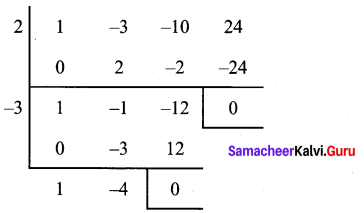Thqs (x – 2) (x + 3) (x – 4) are the factors.
∴ x3 – 3x2 – 10x + 24 = (x – 2) (x + 3) (x – 4)

(ii) 2x2 – 3x2 – 3x + 2
Let p (x) = 2x3 – 3x2 – 3x + 2
Sum of all the co-efficients are
2 – 3 – 3 + 2 = 4 – 6 = -2 ≠ 0
∴ (x – 1) is not a factor
Sum of co-efficients of even powers of x with constant = -3 + 2 = – 1
Sum of co-efficients of odd powers of x = 2- 3= -1
(-1) = (-1)
∴ (x + 1) is a factor
Let us find the other factors using synthetic division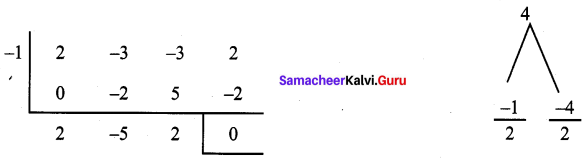Quotient is 2x2 – 5x + 2 = 2x – 4x – x + 2 = 2x (x – 2) – 1 (x – 2)
= (x – 2) (2x – 1)
∴ 2x3 – 3x2 – 3x + 2 = (x + 1) (x – 2) (2x – 1)

(iii) -7x + 3 + 4x3
Let p(x) = 4x3 + 0x2 – 7x + 3
Sum of the co-efficients are = 4 + 0 – 7 + 3
= 7 – 7 = 0
∴ (x- 1) is a factor
Sum of co-efficients of even powers of x with constant = 0 + 3 = 3
Sum of co-efficients of odd powers of x with constant = 4 – 7 = -3
-3 ≠ -3
∴ (x + 1) is not a factor
Using synthetic division, let us find the other factors.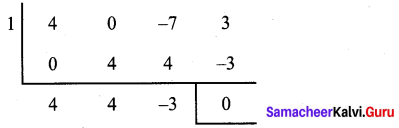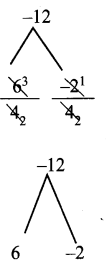Quotient is 4x2 + 4x – 3
= 4x2 + 6x – 2x – 3
= 2x (2x + 3) – 1 (2x + 3)
= (2x + 3) (2x – 1)
∴ The factors are (x – 1), (2x + 3) and (2x – 1)
∴ -7x + 3 + 4x3 = (x + 1) (2x + 3) (2x – 1)(iv) x3 + x2 – 14x – 24
Let p (x) = x3 + x2 – 14x – 24
Sum of the co-efficients are = 1 + 1 – 14 – 24 = -36 ≠ 0
∴ (x – 1) is not a factor
Sum of co-efficients of even powers of x with constant = 1 – 24 = -23
Sum of co-efficients of odd powers of x = 1 – 14 = -3
-23 ≠ -13
∴ (x + 1) is also not a factor
p(2) = 23 + 22 – 14 (2) – 24 = 8 + 4 – 28 – 24
= 12 – 52 ≠ 0, (x – 2) is a not a factor
p (-2) = (-2)3 + (-2)2 – 14 (-2) – 24
= -8 + 4 + 28 – 24 = 32 – 32 = 0
∴ (x + 2) is a factor
To find the other factors let us use synthetic division.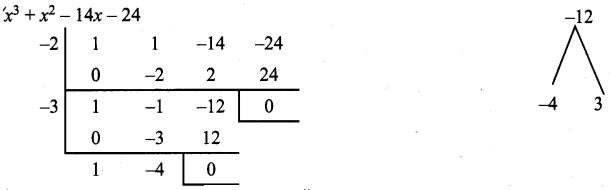∴ The factors are (x + 2), (x + 3), (x + 4)
∴ x3 + x2 – 14x – 24 = (x + 2) (x + 3) (x – 4)

(v) x3 – 7x + 6
Let p (x) = x3 + 0x2 – 7x + 6
Sum of the co-efficients are = 1 + 0 – 7 + 6 = 7 – 7 = 0
∴(x- 1) is a factor
Sum of co-efficients of even powers of x with constant = 0 + 6 = 6
Sum of coefficient of odd powers of x = 1 – 7 = -7
6 ≠ -7
∴ (x + 1) is not a factor
To find the other factors, let us use synthetic division.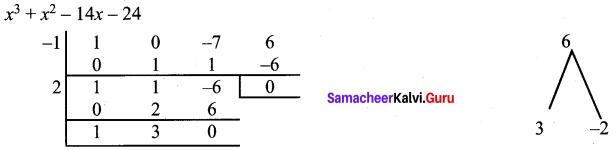∴ The factors are (x – 1), (x – 2), (x + 3)
∴ x3 + 0x2 – 7x + 6 = (x – 1) (x – 2) (x + 3)

(vi) x3 – 10x2 – x + 10
Let p (x) = x3 – 10x2 – x + 10
Sum of the co-efficients = 1 – 0 – 1 + 10
= 11 – 11 = 0
∴ (x – 1) is a factor
Sum of co-efficients of even powers of x with constant = -10 + 10 = 0
Sum of co-efficients of odd powers of = 1 – 1 = 0
∴(x + 1) is a factor
Synthetic division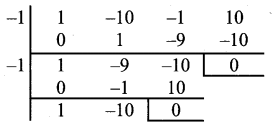∴ x3 + 10x2 – x + 10 = (x – 1) (x + 1) (x – 10)